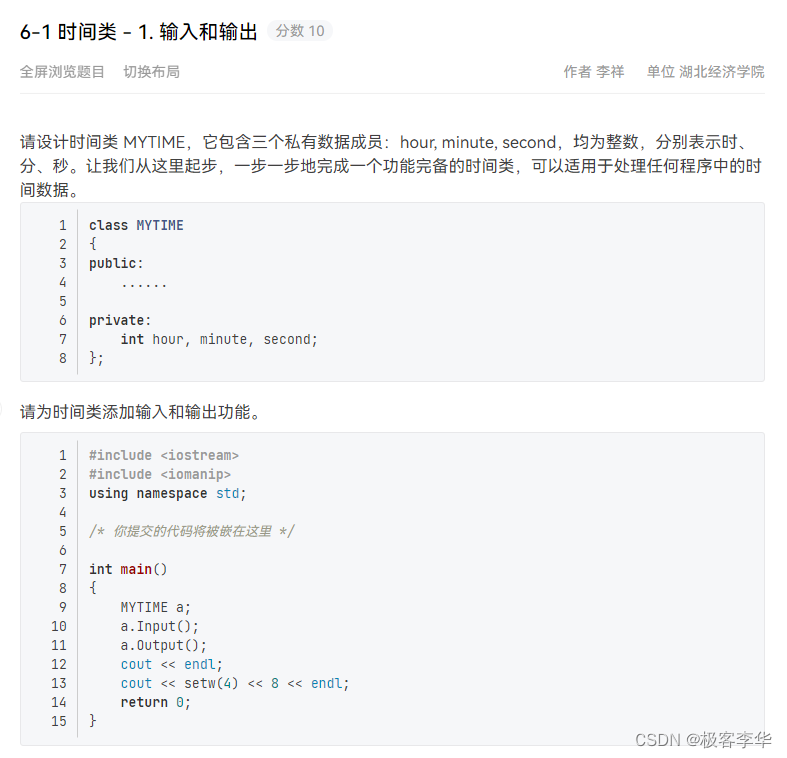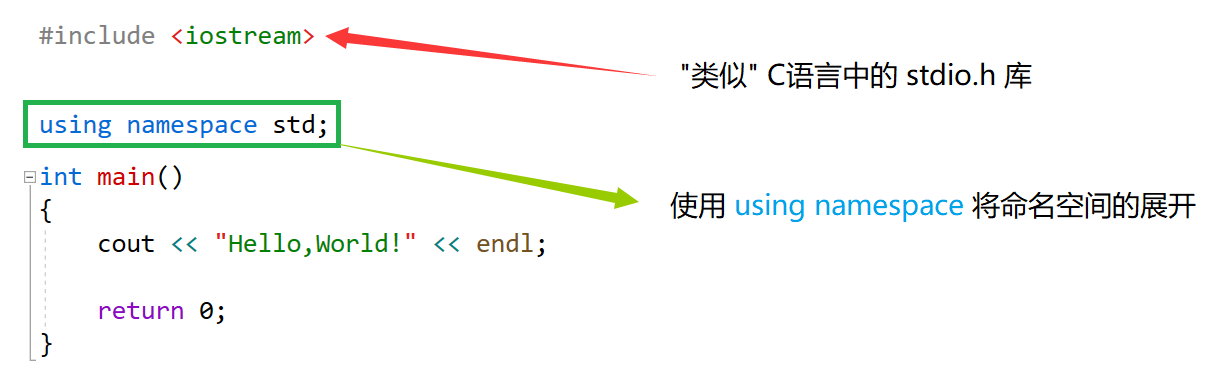# [从C到C++] 1.5 C+的输入与输出

+关注继续查看

cin表示标准输入、cout表示标准输出、cerr表示标准错误

cout是带有缓冲的，而cerr则不带缓冲

[例1]C++简单的输入输出代码示例：

#include<iostream>
using namespace std;
int main()
{
int x;
float y;
cin>>x;
cout<<"The int number is x= "<<x<<endl;
cin>>y;
cout<<"The float number is y= "<<y<<endl;
return 0;
}


8↙
The int number is x= 8
7.4↙
The float number is y= 7.4


[例2]cin 连续输入示例：


#include<iostream>
using namespace std;
int main()
{
int x;
float y;
cout<<"Please input an int number and a float number:"<<endl;
cin>>x>>y;
cout<<"The int number is x= "<<x<<endl;
cout<<"The float number is y= "<<y<<endl;
return 0;
}


Please input an int number and a float number:
8 7.4↙
The int number is x= 8
The float number is y= 7.4


[例3]使用 cin 连续输入数据：

#include<iostream>
using namespace std;
int main()
{
int sum = 0;
int val = 0;
while(cin>>val)
{
sum += val;
}
cout<<"The sum of all number is sum = "<<sum<<endl;
return 0;
}|
11月前
|
C++

154 0|
12月前
|

【C++要笑着学】关键字 | 命名空间 | 输入和输出（二）

86 0|
12月前
|

【C++要笑着学】关键字 | 命名空间 | 输入和输出（一）

81 0|

＜C++＞运算符重载进阶之左移运算符，输出成员属性一步到位
＜C++＞运算符重载进阶之左移运算符，输出成员属性一步到位
169 0
|
C++
C++ 自定义二叉树并输出二叉树图形
C++ 自定义二叉树并输出二叉树图形
157 2
|
C++
C++ 创建链表并输出
C++ 创建链表并输出
129 2